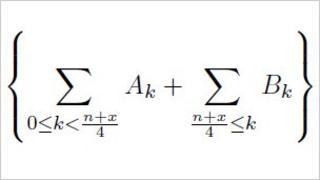# Pi record smashed as team finds two-quadrillionth digitImage caption The formula turns an infinite sum into a more manageable calculation of single terms

A researcher has calculated the 2,000,000,000,000,000th digit of the mathematical constant pi - and a few digits either side of it.

Nicholas Sze, of tech firm Yahoo, said that when pi is expressed in binary, the two quadrillionth "bit" is 0.

Mr Sze used Yahoo's Hadoop cloud computing technology to more than double the previous record.

It took 23 days on 1,000 of Yahoo's computers - on a standard PC, the calculation would have taken 500 years.

The heart of the calculation made use of an approach called MapReduce originally developed by Google that divides up big problems into smaller sub-problems, combining the answers to solve otherwise intractable mathematical challenges.

At Yahoo, a cluster of 1,000 computers implemented this algorithm to solve an equation that plucks out specific "bits" of pi, expressing the constant in binary - the ones and zeroes familiar from computing.

## Pi slicing

The pursuit of longer versions of pi is a long-standing pastime among mathematicians.

But this approach is very different from the full calculation of each digit from the decimal point onward.

Instead, each of the Hadoop computers was working on a formula that turns a complicated equation for pi into a small set of mathematical steps, returning just one, specific piece of pi.

"Interestingly, by some algebraic manipulations, (our) formula can compute pi with some bits skipped; in other words, it allows computing specific bits of pi," Mr Sze explained to BBC News.

This quest for ever-higher binary bits of pi has been ongoing for a number of years, with the former single-digit record held by Colin Percival, founder of the Tarsnap online backup service. He found the quadrillionth bit of pi in 2000.

"The [formula for picking specific digits out] was discovered in 1995, so the fact that there are two separate pi computation records is quite new, historically speaking," Dr Percival told BBC News.

The record for finding consecutive numbers, from 3.14 onward to the final digit, is held by Fabrice Bellard, who announced in January that he had calculated pi to 2.7 trillion digits.

He told BBC News that the single-digit and full pi calculation are vastly different in the degree to which they can be "parallelised" - that is, cut up into manageable pieces among different computers.

He said the current, single-digit record is "more a demonstration of the Hadoop parallelisation framework... it can demonstrate the power of new algorithms which could be useful in other fields".

The record-breaking MapReduce approach, he said, is useful in physics, cryptography and data mining.

Mr Sze added that the calculation was also a good test for the Hadoop hardware and approach.

"This kind of calculation is useful in benchmarking and testing," he said.

"We have used it to compare the [processor] performance among our clusters."

## Related Internet links

The BBC is not responsible for the content of external Internet sites